Ch 4. The Mean Value Theorem Multimedia Engineering Math Maximum & Minimum Rolle'sTheorem Mean ValueTheorem MonotonicFunctions First DerivativeTest Concavity &Inflection SecondDerivative Test
 Chapter 1. Limits 2. Derivatives I 3. Derivatives II 4. Mean Value 5. Curve Sketching 6. Integrals 7. Inverse Functions 8. Integration Tech. 9. Integrate App. 10. Parametric Eqs. 11. Polar Coord. 12. Series Appendix Basic Math Units Search eBooks Dynamics Fluids Math Mechanics Statics Thermodynamics Author(s): Hengzhong Wen Chean Chin Ngo Meirong Huang Kurt Gramoll ©Kurt GramollMATHEMATICS - CASE STUDY SOLUTION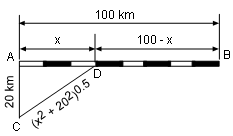Factory Position In order to deliver products to market, a factory which is 20 km away from the railway needs to ship its merchandise to a new railway link D from the highway and then to B railroad. It is known that the total length of the railroad is 100 km and the rate of the unit price to transport through railroad to freeway is 3:5. Where should D be located so that the manufacturer pays the lowest cost for shipping? Let the distance between A and D be x km, then the length of DB equals 100 - x km and CD equals (x2 + 202)0.5 km. It is given that the rate of the unit price to ship through railway to road is 3:5, thus the shipping cost for the railroad and highway per kilometer can be expressed as 3k and 5k respectively. Let the total payment for the transportation be y. The total transportation fee is the sum of the railway and freeway payment.     y = CD(5k) + DB(3k) Substituting DB = 100 - x and CD = (x2 + 202)0.5 into the total payment equation gives     y = 5k(x2 + 202)0.5 + 3k(100 - x) Recall that the minimum payment may occur at the point where the derivative of the function is 0 or does not exist (critical point). In order to find these points, the derivative of the function needs to be calculated.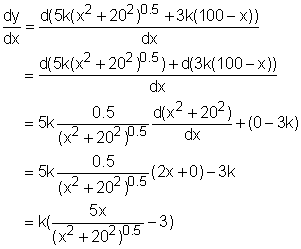As mentioned previously dy/dx needs to be 0 or does not exist. The case, dy/dx does not exist, cannot happen because no matter what value x is, the term (x2 + 202)0.5 cannot be 0. The case, dy/dx equals 0, makes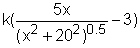to be 0. Since k is a nonzero positive coefficient, x can be found by solving the equation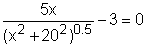. or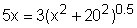Squaring both sides of the equation gives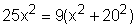Simplifying this equation gives     16x2 = 9(400)     x = 15 or x = -15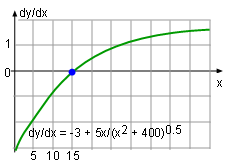Function dy/dx Since x is the distance from A to D, it is larger than 0 and thus the solution, x = -15, is discarded. From the above explanation, x = 15 is the only critical point for the transportation fee function. On the left side of this point, the derivative of the function is less than zero, and on the right side of this point, the derivative of the function is larger than zero as shown on the left graph. This satisfies the test for first derivative function and the value of the function on this point is the minimum value. Overall, the railway link, D, should be built 15 km away from point A.